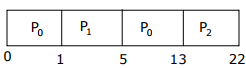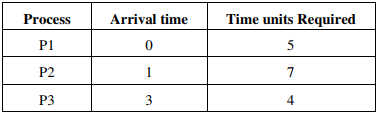# Operating System - Online Test

Q1.  Let the page fault service time be 10ms in a computer with average memory access time being 20ns. If one page fault is generated for every 106 memory accesses, what is the effective access time for the memory?
Explaination / Solution:

P = page fault rate
EA = p × page fault service time
+(1 - p) × Memory access timeQ2. Let the time taken to switch between user and kernel modes of execution be t1 while the time taken to switch between two processes be t2. Which of the following is TRUE?
Explaination / Solution:

Process switching also involves mode changing.

Q3. A computer handles several interrupt sources of which the following are relevant for this question. Interrupt from CPU temperature sensor Interrupt from Mouse Interrupt from Keyboard Interrupt from Hard Disk
Explaination / Solution:
No Explaination.

Q4. Consider the following table of arrival time and burst time for three processes P0, P1 and P2.
Process   Arrival time       Burst Time
P0             0 ms                 9 ms
P1             1 ms                 4ms
P2             2 ms                 9ms
The pre-emptive shortest job first scheduling algorithm is used. Scheduling is carried out only at arrival or completion of processes. What is the average waiting time for the three processes?
Explaination / Solution:Average waiting time = (4+11)/3 = 5 ms

Q5. The amount of ROM needed to implement a 4 bit multiplier is
Explaination / Solution:

For a 4 bit multiplier there are 24 × 24 = 28 = 256 combinations.
Output will contain 8 bits.
So the amount of ROM needed is 28 ×8 bits = 2Kbits

Q6. A process executes the code fork (); fork (); fork (); The total number of child processes created is
Explaination / Solution:

If fork is called n times, there will be total 2n running processes including the parent process. So, there will be 2n -1 child processes.

Q7. A file system with 300 GByte disk uses a file descriptor with 8 direct block addresses, 1 indirect block address and 1 doubly indirect block address. The size of each disk block is 128 Bytes and the size of each disk block address is 8 Bytes. The maximum possible file size in this file system is
Explaination / Solution:

Each block size = 128 Bytes Disk block address = 8 Bytes ∴ Each disk can contain = 128/8 = 16 addresses Size due to 8 direct block addresses: 8 x 128 Size due to 1 indirect block address: 16 x 128 Size due to 1 doubly indirect block address: 16 x 16 x 128 Size due to 1 doubly indirect block address: 16 x 16 x 128 So, maximum possible file size: = 8×128 +16×128 +16×16×128=1024 + 2048 + 32768=35840 Bytes= 35KBytes

Q8. Fetch_And_Add (X, i) is an atomic Read-Modify-Write instruction that reads the value of memory location X, increments it by the value i, and returns the old value of X. It is used in the pseudocode shown below to implement a busy-wait lock. L is an unsigned integer shared variable initialized to 0. The value of 0 corresponds to lock being available, while any nonzero value corresponds to the lock being not available.
AcquireLock(L){
L = 1;
}
Release Lock(L){
L = 0;
}
This implementation

Explaination / Solution:

1. Acquire lock (L) {
3. L = 1.
}
4. Release Lock (L) {
5. L = 0;
6. }
Let P and Q be two concurrent processes in the system currently executing as follows
P executes 1,2,3 then Q executes 1 and 2 then P executes 4,5,6 then L=0 now Q executes 3 by which L will be set to 1 and thereafter no process can set
L to zero, by which all the processes could starve.

Q9. Consider the 3 process, P1, P2 and P3 shown in the tableThe completion order of the 3 processes under the policies FCFS and RR2 (round robin scheduling with CPU quantum of 2 time units) are
Explaination / Solution:

For FCFS Execution order will be order of Arrival time so it is P1,P2,P3 Next For RR with time quantum=2,the arrangement of Ready Queue will be as follows: RQ: P1,P2,P1,P3,P2,P1,P3,P2 This RQ itself shows the order of execution on CPU(Using Gantt Chart) and here it gives the completion order as P1,P3,P2 in Round Robin algorithm.

Q10. Three concurrent processes X, Y, and Z execute three different code segments that access and update certain shared variables. Process X executes the P operation (i.e., wait) on semaphores a, b and c; process Y executes the P operation on semaphores b, c and d; process Z executes the P operation on semaphores c, d, and a before entering the respective code segments. After completing the execution of its code segment, each process invokes the V operation (i.e., signal) on its three semaphores. All semaphores are binary semaphores initialized to one. Which one of the following represents a deadlock-free order of invoking the P operations by the processes?
Explaination / Solution:

Suppose X performs P(b) and preempts, Y gets chance, but cannot do its first wait i.e., P(b), so waits for X, now Z gets the chance and performs P(a) and preempts, next X gets chance. X cannot continue as wait on ‘a’ is done by Z already, so X waits for Z. At this time Z can continue its operations as down on c and d. Once Z finishes, X can do its operations and so Y. In any of execution order of X, Y, Z one process can continue and finish, such that waiting is not circular. In options (A),(C) and (D) we can easily find circular wait, thus deadlock